Atmos. Chem. Phys., 18, 6907–6921, 2018
https://doi.org/10.5194/acp-18-6907-2018
Atmos. Chem. Phys., 18, 6907–6921, 2018
https://doi.org/10.5194/acp-18-6907-2018

Research article 16 May 2018

Research article | 16 May 2018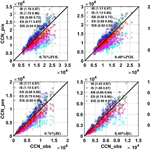# Using different assumptions of aerosol mixing state and chemical composition to predict CCN concentrations based on field measurements in urban Beijing

Using different assumptions of aerosol mixing state and chemical composition to predict CCN concentrations based on field measurements in urban Beijing
Jingye Ren1, Fang Zhang1,2, Yuying Wang1, Don Collins3, Xinxin Fan1, Xiaoai Jin1, Weiqi Xu4,5, Yele Sun4,5, Maureen Cribb6, and Zhanqing Li1,6 Jingye Ren et al.
• 1College of Global Change and Earth System Science, Beijing Normal University, Beijing 100875, China
• 2Joint Center for Global Change Studies (JCGCS), Beijing 100875, China
• 3Department of Atmospheric Sciences, Texas A & M University, College Station, TX, USA
• 4State Key Laboratory of Atmospheric Boundary Layer Physics and Atmospheric Chemistry, Institute of Atmospheric Physics, Chinese Academy of Sciences, Beijing 100029, China
• 5University of Chinese Academy of Sciences, Beijing 100049, China
• 6Earth System Science Interdisciplinary Center and Department of Atmospheric and Oceanic Science, University of Maryland, College Park, MD, USA

Correspondence: Fang Zhang (fang.zhang@bnu.edu.cn)

Abstract

Understanding the impacts of aerosol chemical composition and mixing state on cloud condensation nuclei (CCN) activity in polluted areas is crucial for accurately predicting CCN number concentrations (NCCN). In this study, we predict NCCN under five assumed schemes of aerosol chemical composition and mixing state based on field measurements in Beijing during the winter of 2016. Our results show that the best closure is achieved with the assumption of size dependent chemical composition for which sulfate, nitrate, secondary organic aerosols, and aged black carbon are internally mixed with each other but externally mixed with primary organic aerosol and fresh black carbon (external–internal size-resolved, abbreviated as EI–SR scheme). The resulting ratios of predicted-to-measured NCCN (RCCN_p∕m) were 0.90 – 0.98 under both clean and polluted conditions. Assumption of an internal mixture and bulk chemical composition (INT–BK scheme) shows good closure with RCCN_p∕m of 1.0 –1.16 under clean conditions, implying that it is adequate for CCN prediction in continental clean regions. On polluted days, assuming the aerosol is internally mixed and has a chemical composition that is size dependent (INT–SR scheme) achieves better closure than the INT–BK scheme due to the heterogeneity and variation in particle composition at different sizes. The improved closure achieved using the EI–SR and INT–SR assumptions highlight the importance of measuring size-resolved chemical composition for CCN predictions in polluted regions. NCCN is significantly underestimated (with RCCN_p∕m of 0.66 – 0.75) when using the schemes of external mixtures with bulk (EXT–BK scheme) or size-resolved composition (EXT–SR scheme), implying that primary particles experience rapid aging and physical mixing processes in urban Beijing. However, our results show that the aerosol mixing state plays a minor role in CCN prediction when the κorg exceeds 0.1.

Share
1 Introduction

Atmospheric aerosol particles can serve as cloud condensation nuclei (CCN) and, in turn, affect the optical and microphysical properties of clouds (Twomey, 1977; Albrecht, 1989; Charlson et al., 1992). Additionally, an increase in the aerosol number concentration may suppress precipitation in shallow clouds and promote it in deep convective clouds (Rosenfeld et al., 2008; Li et al., 2011). A key challenge to understanding indirect aerosol effects is quantifying CCN spectra and their spatial and temporal variations.

The ability of particles to act as CCN mainly depends on their size, chemical composition, and mixing state (McFiggans et al., 2006; Dusek et al., 2006; Ma et al., 2013). The impacts of the size distribution and chemical composition on CCN activity has been discussed in previous studies (Dusek et al., 2006; Ervens et al., 2007; Broekhuizen et al., 2006; Yum et al., 2005, 2007; Wiedensohler et al., 2009; Deng et al., 2013; Zhang et al., 2014, 2016; Kawana et al., 2016). The effect of chemical composition can be represented by a hygroscopicity parameter (κ) (Petters and Kreidenweis, 2007) which is often used to predict NCCN (Moore et al., 2012; Zhang et al., 2014). However, particle composition may vary from single species to a mixture of multiple species for a given size. A description of size-resolved chemical composition thus leads to a better prediction of NCCN because it allows variation of κ with size (Medina et al., 2007; Wang et al., 2010; Meng et al., 2014). Variations in mixing state also impact NCCN prediction, with the effect dependent on the hygroscopicity of the organic component (Wang et al., 2010). The assumption of internal mixtures has been demonstrated to predict NCCN well (Ervens et al., 2007; Chang et al., 2007; Andreae and Rosenfeld, 2008; Gunthe et al., 2009; Rose et al., 2008; Meng et al., 2014; Zhang et al., 2014; Li et al., 2017). However, some studies have shown that detailed information about the chemical composition and the mixing state is required because of the complexity of the hygroscopicity of organics (Broekhuizen et al., 2006; Bhattu and Tripathi, 2015) and the differences in the CCN activity between fresh and aged aerosols (Gunthe et al., 2011). Therefore, the impact of different assumptions concerning the mixing state and chemical composition on accurately quantifying CCN concentrations needs further investigation, especially in heavily polluted regions.

Beijing, a typical polluted city, frequently experiences severe haze pollution episodes (Sun et al., 2013; Guo et al., 2014; Zheng et al., 2015), particularly in winter. Several recent studies have focused on studying particle hygroscopicity (Wu et al., 2016; Wang et al., 2017) and chemical composition (Gunthe et al., 2011), and using bulk κ to predict CCN in Beijing (e.g., Liu et al., 2014; Zhang et al., 2017). However, to our knowledge, a comprehensive CCN closure test considering chemical composition and mixing state is lacking for this polluted urban area. In particular, the transformation of the particle mixing state may be very quick during severe pollution conditions (Wu et al., 2016). During pollution events, the hygroscopicity of organics and CCN activity are often enhanced rapidly with the aging process (Gunthe et al., 2011; Kawana et al., 2016). Therefore, the characterization and parameterization of CCN activation may be more challenging in polluted regions due to the impacts of organics (Wang et al., 2010; Meng et al., 2014; Che et al., 2016; Zhang et al., 2016).

In this study, we use size-resolved measurements of CCN activity and size-resolved chemical composition information to predict NCCN using field measurement data collected in Beijing during the winter of 2016. The CCN closure study is carried out using five schemes with different assumptions regarding particle mixing state and chemical composition. By classifying the data into three different periods (nighttime, noontime, and the evening rush hour), we also investigate the variations in aerosol mixing state from fresh to relatively aged aerosols. The sensitivity of predicted NCCN to the particle mixing state and organic volume fraction with the aging of organic particles is also presented in the last section of the study.

2 Measurements and data

Data used here were measured from 15 November to 14 December 2016 during the Air Pollution and Human Health (APHH) field campaign at the Institute of Atmospheric Physics (IAP), Chinese Academy of Sciences (39.97 N, 116.37 E), which is a typical urban site with influences from traffic and cooking emissions (Sun et al., 2015). The sampling instruments were placed in a container at ground level.

The particle number size distribution (PNSD) was measured by a scanning mobility particle sizer (SMPS; Wang et al., 2003). The SMPS consists of a differential mobility analyzer (DMA; model 3081, TSI Inc.) and a condensation particle counter (CPC; model 3772, TSI Inc.). Measurements of size-resolved CCN efficiency spectra were made by an integrated system combining the SMPS (Wang et al., 2003) and a Droplet Measurement Technologies CCN counter (DMT-CCNc; Lance et al., 2006). The procedure to couple the SMPS and the DMT-CCNc developed by Moore et al. (2010) was followed. Atmospheric particles were sampled from an inlet located 1.5 m above the roof of the container and were then passed through a silica gel desiccant drying tube into the SMPS. The relative humidity of the sample flow was below 30 %. The sample flow exiting the DMA was divided into 0.5 L min−1 for the CCNc and 0.5 L min−1 for the CPC. Before and after the field campaign ammonium sulfate was used to calibrate the supersaturation (SS) levels of the CCNc with longitudinal temperature differences of 2, 3, 5, 8, 10, 13, and 15 K as shown in Fig. S1 in the Supplement. Based on this calibration, the five effective SS levels were 0.12, 0.14, 0.23, 0.40, and 0.76 %.

The PNSD spanned the size range of 10 – 550 nm with a measurement scan time of 5 min. Total particle or condensation nuclei (CN) size distributions were calculated with the multiple charge correction and transfer function used in the TSI-AIM software. The CN number concentration (NCN) is the total aerosol number concentration and is obtained by integrating the PNSD over the size range of 10 – 550 nm. The full measurement cycle of the CCNc for the five SS levels took one hour (20 min for 0.12 % and 10 min for each higher SS). Size-resolved CCN efficiency data were inverted with a multiple charge correction (Moore et al., 2010). The CCN number size distribution was calculated by multiplying the CCN efficiency spectrum by the particle number size distribution. The total CCN concentration was then calculated by integrating the size-resolved NCCN. The bulk activation ratio (AR) was calculated as NCCNNCN. The results were stratified between polluted and background conditions with an assumed threshold PM1 mass concentration of 50 µg m−3.

An Aerodyne high-resolution time-of-flight aerosol mass spectrometer (HR-ToF-AMS; DeCarlo et al., 2006) was housed in a sampling room on the rooftop of a two-story building to measure size-resolved non-refractory submicron aerosols, including organics, sulfate, nitrate, ammonium, and chloride with a time resolution of  5 min. More details about the HR-ToF-AMS and the measurement site have been described in previous studies (Sun et al., 2010; Sun et al., 2016). The organics are classified using positive matrix factorization (PMF) (Paatero and Tapper, 1994), considering as being composed of two components: primary organic aerosol (POA) representing non-hygroscopic particles and secondary organic aerosol (SOA) representing hygroscopic particles. The first component consists mainly of hydrocarbon-like organic aerosol (HOA), a surrogate of primary organic aerosol (POA) from local combustion sources. The size distribution of the primary organic aerosol (OA) was measured by the estimated size-distribution of the C4H${}_{\mathrm{9}}^{+}$ fragment (Aiken et al., 2009; Zhang et al., 2005). The size distribution of the SOA was calculated as the difference between the total OA and POA.

The black carbon (BC) mass concentration was measured using a seven-wavelength aethalometer (AE33, Magee Scientific Corp.). Zhao et al. (2017) provides details about this instrument and the measurements it makes. Due to an absence of size-resolved BC measurements, the BC size distribution was calculated from the combination of an approximately lognormal distribution measured by a single particle soot photometer (SP2, DMT) (Wu et al., 2017) and the total BC mass concentration. Note that because the SP2 measures BC core diameter instead of the diameter of the BC-containing particle, it would overestimate the BC mass concentration of smaller particles but underestimate that of larger particles. Such overestimation would likely lead to an underestimation of NCCN due to the increased mass fraction of BC of total particles. The uncertainty of this effect is evaluated in Sect. 4.3. The fresh and aged BC size distributions are determined from the total BC size distribution measured by the SP2 (Wu et al., 2017) and from the dependence of the fraction of internally mixed soot (Fin) on particle diameter (Dp) observed in urban Beijing by Cheng et al. (2012); the instruments produce different diameters. In this paper, we have unified both the aerodynamic diameter from AMS and volume equivalent diameter from SP2 to be mobility diameter. In addition, actual fresh BC particles are not spheres and neither are some of the partially aged BC, but because both the diameter measured from SP2 and the BC size distribution from the literature assume these particles are spheres, the fresh and aged BC in this study are therefore also assumed to be spherical.

3 Theory

## 3.1 Calculation of CCN concentration using κ-Köhler theory

In this study, we used the critical or cutoff particle diameter (Dcut) and particle number size distribution to calculate NCCN. The method to derive Dcut is based on κ-Köhler theory (Petters and Kreidenweis, 2007), with the water vapor saturation ratio over the aqueous solution droplet S given by the following:

$\begin{array}{}\text{(1)}& S=\frac{{D}^{\mathrm{3}}-{D}_{\mathrm{p}}^{\mathrm{3}}}{{D}^{\mathrm{3}}-{D}_{\mathrm{p}}^{\mathrm{3}}\left(\mathrm{1}-\mathit{\kappa }\right)}\mathrm{exp}\left(\frac{\mathrm{4}{\mathit{\sigma }}_{\mathrm{w}}{M}_{\mathrm{w}}}{RT{\mathit{\rho }}_{\mathrm{w}}D}\right),\end{array}$

where D is the droplet diameter, Dp is the dry diameter of the particle, Mw is the molecular weight of water, σw is the surface tension of pure water, ρw is the density of water, R is the gas constant, and T is the absolute temperature. When κ> 0.1 it can be approximately expressed as

$\begin{array}{}\text{(2)}& & \mathit{\kappa }=\frac{\mathrm{4}{A}^{\mathrm{3}}}{\mathrm{27}{D}_{\mathrm{p}}^{\mathrm{3}}{\mathrm{ln}}^{\mathrm{2}}{S}_{\mathrm{c}}},\text{(3)}& & A=\frac{\mathrm{4}{\mathit{\sigma }}_{\mathrm{w}}{M}_{\mathrm{w}}}{RT{\mathit{\rho }}_{\mathrm{w}}},\end{array}$

where Sc is the particle critical supersaturation. The other variables in the equations are T= 298.15 K, R= 8.315 J K−1 mol−1, ρw= 997.1 kg m−3, Mw= 0.018015 kg mol−1, and σw= 0.072 J m−2 (Rose et al., 2008).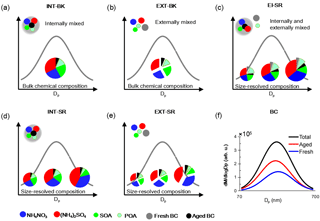Figure 1Schematic representation of the five different schemes: (a) INT–BK, (b) EXT–BK, (c) EI–SR, (d) INT–SR, and (e) EXT–SR, in addition to  (f) the BC size distribution used in this study. The fresh and aged BC size distribution are retrieved from the total BC size distribution measured by the SP2 (Wu et al., 2017) and the dependence of the fraction of internally mixed soot (Fin) on particle diameter (Dp) observed in urban Beijing (Cheng et al., 2012). The total BC size distribution is used in the INT–SR and EXT–SR schemes, and the aged and fresh BC distributions are used in the EI–SR scheme. In the EI–SR scheme, some BC particles are assumed to already be aged and thus internally mixed with sulfate, nitrate, and SOA, and some of them together with POA are freshly emitted and assumed not yet aged/coated by other species (externally mixed).

For internally mixed particles, κ is calculated as follows (Petters and Kreidenweis, 2007; Gunthe et al., 2009):

$\begin{array}{}\text{(4)}& & {\mathit{\kappa }}_{\mathrm{chem}}=\sum _{i}{\mathit{\epsilon }}_{i}{\mathit{\kappa }}_{i},\text{(5)}& & {\mathit{\kappa }}_{\mathrm{org}}={f}_{\mathrm{POA}}\cdot {\mathit{\kappa }}_{\mathrm{POA}}+{f}_{\mathrm{SOA}}\cdot {\mathit{\kappa }}_{\mathrm{SOA}},\end{array}$

where κi and εi are the hygroscopicity parameter and volume fraction for the individual components in the mixture, respectively, and fPOA and fSOA are the primary organic aerosol (POA) and secondary organic aerosol (SOA) mass fractions in the mixture, respectively. The aerosol mass spectrometer (AMS) mainly measured the particle mass size distributions of SO${}_{\mathrm{4}}^{\mathrm{2}-}$, NO${}_{\mathrm{3}}^{-}$, NH${}_{\mathrm{4}}^{+}$, and organic compounds, while the Zdanovskii–Stokes–Robinson relation requires the volume fractions of the particle chemical composition (Stokes and Robinson, 1966; Zdanovskii, 1948). A simplified ion pairing scheme is used to calculate the mass concentrations of the inorganic salts, which includes only NH4NO3 and (NH4)2SO4 as possible salts (Gysel et al., 2007). In this study, we considered five components: NH4NO3, (NH4)2SO4, SOA, POA, and BC. The ${\mathit{\kappa }}_{\left({\mathrm{NH}}_{\mathrm{4}}{\mathrm{NO}}_{\mathrm{3}}\right)}$ is equal to 0.67 and ${\mathit{\kappa }}_{\left(\left({\mathrm{NH}}_{\mathrm{4}}\right)\mathrm{2}{\mathrm{SO}}_{\mathrm{4}}\right)}$ is equal to 0.61 (Petters and Kreidenweis, 2007; Gunthe et al., 2009). The κorg is estimated using the linear function derived by Mei et al. (2013a), namely, κorg= 2.10f44 0.11, where f44 is dependent upon organics oxidation level. The mean κorg is 0.10 in our case. The organics are classified into two factors: POA representing non-hygroscopic particles (κ= 0) and SOA representing hygroscopic species. In our study, the average contributions of POA and SOA to total organics were 0.53 and 0.47, respectively. On the basis of Eq. (5), κ(SOA) is assumed to be 0.2. κ(BC) is furthermore assumed to be 0.

## 3.2 Assumptions about mixing state and chemical composition

To examine the influence of the mixing state and chemical composition on CCN activation, five assumptions (Fig. 1) are used to predict NCCN. Although the assumption of completely internal or external mixing for ambient aerosols represents two extremely simplified schemes and may be atmospherically unrealistic, it allows us to understand the importance of the particle mixing state for predicting NCCN. In addition, size independent and dependent compositions are derived from the mass concentrations of different species measured by the AMS so that the impact of chemical composition on CCN activity can be examined. A detailed introduction of the five assumption schemes follows.

### 3.2.1 Assumption 1: internal mixture with bulk chemical composition (INT–BK)

In this scheme, submicron particles are assumed to be internally mixed with bulk chemical composition, where the mass fraction of each component (e.g., NH4NO3, (NH4)2SO4, SOA, POA, and BC) is uniform throughout the full size range as shown in Fig. 1a. The overall κis calculated from the bulk chemical composition measured by the AMS based on the simple mixing rule (Eq. 4) to obtain the critical diameter at a given SS. For calculating NCCN all (and only) particles with diameters greater than Dcut are considered CCN-active. The total NCCN is then calculated from the step-wise integration of the PNSD for Dp>Dcut. The equations used in the calculations are as follows:

$\begin{array}{}\text{(6)}& {\mathrm{CCN}}_{\mathrm{pre}}=\underset{{D}_{\mathrm{cut}}}{\overset{{D}_{\mathrm{end}}}{\int }}n\left(\mathrm{log}{D}_{\mathrm{p}}\right)d\mathrm{log}{D}_{\mathrm{p}}\end{array}$
$\begin{array}{}\text{(7)}& {D}_{\mathrm{cut}}=\sqrt[\mathrm{3}]{\frac{\mathrm{4}{A}^{\mathrm{3}}}{\mathrm{27}\sum _{i}{\mathit{\epsilon }}_{i}{\mathit{\kappa }}_{i}{\mathrm{ln}}^{\mathrm{2}}{S}_{\mathrm{c}}}},\end{array}$

where Dcut is the critical diameter, Dend is the upper size limit of the PNSD, n (log Dp) is the function of the aerosol number size distribution, i is the chemical component element, and the other parameters are the same as those presented in Eqs. (2)–(4).

### 3.2.2 Assumption 2: internal mixture with size-resolved chemical composition (INT–SR)

For this scheme submicron particles are assumed to be internally mixed and the chemical composition is size-dependent as shown in Fig. 1d. The fractional contributions of the components at each size bin are derived from mass size distributions of the five species considered, i.e., NH4NO3, (NH4)2SO4, SOA, POA, and BC.

For this assumption, the critical diameter is derived from the total hygroscopic parameter, κ, at each size bin, j. For each size bin for which Dp,j is greater than the calculated Dcut,j the activated fraction was assumed to be 1.0 and for all others it was 0.0. The NCCN is calculated as follows:

$\begin{array}{}\text{(8)}& & {\mathrm{CCN}}_{\mathrm{pre}}=\underset{{D}_{\mathrm{begin}}}{\overset{{D}_{\mathrm{end}}}{\int }}n\left(\mathrm{log}{D}_{\mathrm{p}}\right)d\mathrm{log}{D}_{\mathrm{p}}\text{(9)}& & {D}_{\mathrm{cut},j}=\sqrt[\mathrm{3}]{\frac{\mathrm{4}{A}^{\mathrm{3}}}{\mathrm{27}\sum _{i}{\mathit{\epsilon }}_{ij}{\mathit{\kappa }}_{ij}{\mathrm{ln}}^{\mathrm{2}}{S}_{\mathrm{c}}}},\end{array}$

where Dbegin and Dend are the first and last diameters of the PNSD, n (log Dp) is the function of the aerosol number size distribution, i is the chemical component element, j is the PNSD size bin, and the other parameters are the same as those presented in Eqs. (2)–(4).

### 3.2.3 Assumption 3: external mixture with bulk chemical composition (EXT–BK)

For this scheme the submicron aerosol is treated as an external mixture. This means that there are five types of particles, i.e., NH4NO3, (NH4)2SO4, SOA, POA, and BC, and each particle consists of a single component. The volume fraction of each component, which is derived from bulk mass concentrations, does not vary with size (as shown in Fig. 1b).

At a given S, the critical diameter of each particle type is retrieved from the κ of each component. The NCCN of each aerosol type is calculated as the CCN-active particle number concentration multiplied by the bulk volume fraction of the components as expressed in Eq. (10). The NCCN of the five particle types are finally summed to obtain the total NCCN. The specific equations are as follows:

$\begin{array}{}\text{(10)}& & {\mathrm{CCN}}_{\mathrm{pre}}=\sum _{i}\left(\underset{{D}_{\mathrm{cut},i}}{\overset{{D}_{\mathrm{end}}}{\int }}n\left(\mathrm{log}{D}_{\mathrm{p}}\right)d\mathrm{log}{D}_{\mathrm{p}}\cdot {V}_{i}\right)\text{(11)}& & {D}_{\mathrm{cut},i}=\sqrt[\mathrm{3}]{\frac{\mathrm{4}{A}^{\mathrm{3}}}{\mathrm{27}{\mathit{\kappa }}_{i}{\mathrm{ln}}^{\mathrm{2}}{S}_{\mathrm{c}}}},\end{array}$

where Dcut,i is calculated for each component, i, at a given SS, Vi is the volume fraction of each aerosol type, n (log Dp) is the function of the aerosol number size distribution, i is the chemical component element, and the other parameters are the same as those presented in Eqs. (2)–(4).

### 3.2.4 Assumption 4: external mixture with size-resolved chemical composition (EXT–SR)

As with the EXT–BK scheme the same five particle types are considered and their relative concentrations selected to match the measured composition. But unlike with the EXT–BK scheme the relative concentrations of the five particle types vary with particle size to capture the size-dependence of the measured composition, as is depicted in Fig. 1e. The volume fraction of each particle type at each size is first multiplied by the total particle number size distribution (PNSD) to get the PNSDi of each aerosol type. The NCCN of each particle type is then obtained from the step-wise integration of the PNSDi for Dp>Dcut,i, and summed to get the total NCCN, as described by Eq. (12). Similar to EXT–BK, the critical diameter of each particle type is also derived from the κ of each pure component at a given S.

$\begin{array}{}\text{(12)}& & {\mathrm{CCN}}_{\mathrm{pre}}=\sum _{i}\left(\underset{{D}_{\mathrm{begin}}}{\overset{{D}_{\mathrm{end}}}{\int }}\left(n\left(\mathrm{log}{D}_{\mathrm{p}}\right)\cdot {V}_{ij}\right)d\mathrm{log}{D}_{\mathrm{p}}\right)\text{(13)}& & {D}_{\mathrm{cut},i}=\sqrt[\mathrm{3}]{\frac{\mathrm{4}{A}^{\mathrm{3}}}{\mathrm{27}{\mathit{\kappa }}_{i}{\mathrm{ln}}^{\mathrm{2}}{S}_{\mathrm{c}}}},\end{array}$

where Vi is the volume fraction of each particle type in a size bin, n (log Dp) is the function of the aerosol number size distribution, i is the chemical component element, j is the particle size bin, and the other parameters are the same as those presented in Eqs. (2)–(4).

### 3.2.5 Assumption 5: sulfate, nitrate, SOA, and aged BC internally mixed, and POA and fresh BC externally mixed, and all components with size-resolved chemical composition (EI–SR)

At each particle size sulfate, nitrate, and SOA with BC-aged are treated as internally mixed, but POA and BC-fresh are present in separate particles and are non-hygroscopic. As with INT–SR and EXT–SR the chemical composition is size-dependent, as shown in Fig. 1c. The EI–SR scheme likely represents a case that is most similar to that of actual atmospheric aerosols in locations such as Beijing. The fresh and aged BC size distributions are determined from the total BC size distribution measured by the SP2 (Wu et al., 2017) and from the dependence of the fraction of internally mixed soot (Fin) on particle diameter (Dp) observed in urban Beijing by Cheng et al. (2012).

In this assumption the fresh BC and POA particles can serve as CCN only if their diameter is larger than 200 nm – otherwise they are CCN-inactive. Thus, the total NCCN of those externally mixed components (NCCN_EXT) is calculated from the step-wise integration of the product of the PNSD and the volume fraction of the fresh BC and POA in each size bin larger than 200 nm.

The NCCN of the remaining components (sulfate, nitrate, and SOA with BC-aged) that are treated as an internal mixture, denoted as NCCN_INT, is predicted in the same way as for the INT–SR scheme, with the only difference being that the PNSD is first multiplied by the volume fraction of the mixed component particles for each size bin. The total NCCN is thus calculated as the sum of NCCN_EXT and NCCN_INT. The specific equations are as follows:

$\begin{array}{ll}{\mathrm{CCN}}_{\mathrm{pre}}& =\underset{{D}_{\mathrm{begin}}}{\overset{{D}_{\mathrm{200}}}{\int }}\left(n\left(\mathrm{log}{D}_{\mathrm{p}}\right)\cdot {r}_{j}\right)d\mathrm{log}{D}_{\mathrm{p}}\\ \text{(14)}& & +\underset{{D}_{\mathrm{200}}}{\overset{{D}_{\mathrm{end}}}{\int }}n\left(\mathrm{log}{D}_{\mathrm{p}}\right)d\mathrm{log}{D}_{\mathrm{p}}\end{array}$

$\begin{array}{}\text{(15)}& {D}_{\mathrm{cut},j}=\sqrt[\mathrm{3}]{\frac{\mathrm{4}{A}^{\mathrm{3}}}{\mathrm{27}\sum _{i}{\mathit{\epsilon }}_{ij}{\mathit{\kappa }}_{ij}{\mathrm{ln}}^{\mathrm{2}}{S}_{\mathrm{c}}}},\end{array}$

where Dbegin and Dend are the first and last diameters of the PNSD, n (log Dp) is the function of the aerosol number size distribution, r is the volume fraction of the internal (hygroscopic) mixture at each size, i is the chemical component element, j is the particle size bin, and the other parameters are the same as those presented in Eqs. (2)–(4).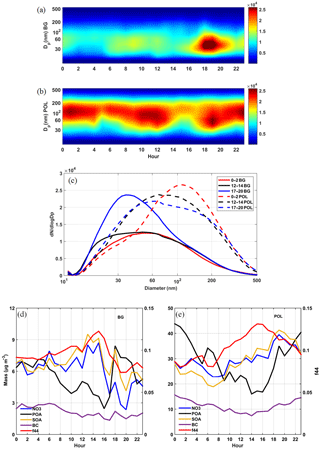Figure 2Diurnal variations in aerosol properties at the IAP site during the APHH field experiment, including the particle number size distribution measured by the SMPS under (a) background (BG) and (b) polluted (POL) conditions. (c) Mean particle number size distribution measured by the SMPS during three periods (00:00–02:00, 12:00–14:00, and 17:00–20:00 LT) under BG and POL conditions. Bulk chemical component mass concentrations (NO3, POA, SOA, and BC) and f44 made under (d) BG and (e) POL conditions.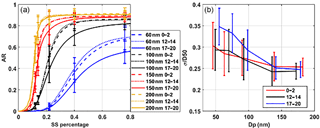Figure 3(a) Averaged fitted CCN efficiency spectra during the nighttime period (00:00–02:00 LT, dashed lines), the noontime period (12:00–14:00 LT, dotted lines), and the evening rush hour period (17:00–20:00 LT, solid lines) for different diameters (60, 100, 150, and 200 nm). (b) The heterogeneity of aerosol particles (σaDa) derived from Eq. (17) during the three selected periods.

4 Results and discussion

## 4.1 Diurnal variations in aerosol properties

Diurnal variations in mean PNSD and bulk chemical composition under polluted and background conditions are shown in Fig. 2. Significant diurnal variations in PNSD are observed during the campaign. For both polluted and background cases the abrupt increases in concentration of small particles (Dp< 100 nm) from 17:00 to 20:00 LT (local time) are likely related to fresh primary emissions from cooking and traffic sources (Wang et al., 2017; Zhao et al., 2017), which is also evident in the significant increase in mass concentration of non-hygroscopic POA (Fig. 2d and e). The peak amplitude in the PNSD that occurs from about 08:00 to 12:00 LT is probably associated with secondary formation processes, which is indicated by an apparent increase of nitrate, SOA, and f44 (oxidation level of organics) in the morning (08:00 LT) when photochemistry becomes significant. The effect is more apparent on clean days. In addition, the PNSD amplitude and BC and POA concentrations are high at nighttime, suggesting an influence from the diurnal variation of the planetary boundary layer (PBL) height. In particular, on polluted days the PBL plays a key role in regulating the diurnal variation of primary components like POA and BC (e.g., Dzepina et al., 2009; Cross et al., 2009). On clean days secondary formation and primary sources play dominant roles in regulating diurnal variations. The PNSD in clean cases has peaks at smaller Dp ( 30–40 nm, Fig. 1c) compared to polluted cases ( 100 nm), which is associated with particle growth accompanying atmospheric chemistry processes during haze evolution (Guo et al., 2014; Wang et al., 2016).

## 4.2 Cumulative Gaussian distribution function fit and parameters derived from the CCN efficiency

The activated fractions measured at the five supersaturation levels were fitted using the following two functions (Rose et al., 2008; Mei et al., 2013b):

$\begin{array}{}\text{(16)}& & {R}_{\mathrm{a}}\left(S\right)=\frac{E}{\mathrm{2}}\cdot \left(\mathrm{1}+\mathrm{erf}\left(\frac{\mathrm{ln}S-\mathrm{ln}{S}^{*}}{\sqrt{\mathrm{2}{\mathit{\sigma }}_{\mathrm{s}}^{\mathrm{2}}}}\right)\right),\text{(17)}& & {f}_{{N}_{\mathrm{CCN}}/{N}_{\mathrm{CN}}}=a\left(\mathrm{1}+\mathrm{erf}\left(\frac{D-{D}_{\mathrm{a}}}{{\mathit{\sigma }}_{\mathrm{a}}\sqrt{\mathrm{2}}}\right)\right),\end{array}$

where Ra(S) and f(NCCN∕NCN) are the CCN activation fractions, the maximum activation fraction (MAF) is equal to E or 2a, S* and Da are the midpoint activation supersaturation and diameter, respectively, and σs and σa are the cumulative distribution function (CDF) standard deviations. During this field campaign, 2580 size-resolved CCN efficiency spectra at five SS levels were measured. To illustrate the characteristics of the activation spectra, the CDF fits are shown in Fig. 3 and in Tables S1 and S2 in the Supplement. A gradual increase in size-resolved AR with SS suggests that particles had different hygroscopicities even at the same diameter. The heterogeneity of particle chemical composition can be represented by the ratio of σa and Da (i.e., σaDa), where σa is the standard deviation derived from the cumulative Gaussian distribution function (Eq. 17) and Da is the activation diameter (Rose et al., 2010). The ratio of σaDa during the three periods is shown in Fig. 3b.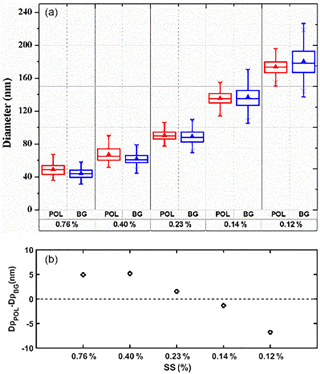Figure 4(a) Retrieved mean critical activation diameters at SS = 0.12, 0.14, 0.23, 0.40, and 0.76 % under background (BG) and polluted (POL) conditions. The box plots show mean critical activation diameters at the 25th, 50th, and 75th percentiles. (b) Difference in the mean critical activation diameter between BG and POL cases.

### 4.2.1 CCN activation curves and heterogeneity of chemical components

For larger particles with Dp> 100 nm, no significant differences were observed in the CCN efficiency spectra (Fig. 3a), suggesting a similar hygroscopicity during the three periods. For particles with a Dp< 100 nm, the CCN efficiency spectrum observed during the evening rush hour period showed a much more gradual increase (with smaller slopes) in size-resolved AR than that derived for the other two periods. This is attributed to the strong influence of POA emissions, which consist of less hygroscopic and externally mixed smaller particles mainly from cooking and traffic during the evening rush hour period (also indicated by the increased σaDa). Particles with a Dp< 100 nm emitted during the evening rush hour period require a higher SS to reach the same AR. However, for a Dp> 100 nm the slope of AR with respect to SS was steep and near the instrumental limit obtained for a pure ammonium sulfate aerosol. Che et al. (2016) have reported that particles larger than about 150 nm have relatively uniform composition. This suggests that particles become more internally mixed with growth from the Aitken mode to the accumulation mode. This feature is also suggested by the decreasing σaDa with increasing particle diameter.

### 4.2.2 Mean critical activation diameter

The critical activation diameter at different SS levels under background and polluted conditions is shown in Fig. 4. The differences in critical diameter between polluted and background cases are calculated as Dp_POLDp_BG. At lower SS levels the critical diameters for polluted cases were slightly smaller than those observed on clean days, suggesting larger particles are more CCN-active on polluted days. This is expected based on a hygroscopic tandem differential mobility analyzer (HTDMA) measurements that showed that particles in the accumulation mode on polluted days are more hygroscopic than those on clean days in urban Beijing (Wang et al., 2017). At higher SS the critical diameter on polluted days was a little higher than that obtained under clean conditions, suggesting that particles with a Dp of  40 nm are less CCN active. This is likely because a high concentration of small and hygroscopic particles in the Aitken mode arise from the photochemistry-driven nucleation process on clean days. However, in polluted cases, small particles are mostly composed of hydrophobic POA from cooking and traffic sources. This was also observed by Wang et al. (2017) who showed that 40 nm particles are less hygroscopic on polluted days. However, the differences in critical diameter between polluted and background cases are small, reflecting a relatively minor influence of hygroscopicity on CCN activity.

### 4.2.3 Maximum activation fraction (MAF)

As shown in Fig. 5, the maximum activated fractions on clean and polluted days during the campaign are less than 1, which suggests that at least some sampled aerosols were externally mixed (Gunthe et al., 2011). For example, the MAF for particles with a Dp of  180 nm was around 0.78 at SS = 0.12 % under background conditions, indicating that  22 % of the particles are non-hygroscopic. The higher MAFs under polluted conditions suggest a more internally mixed aerosol (Wu et al., 2016; Wang et al., 2017). The MAF during the 12:00–14:00 LT period was highest, which is likely due to strong photochemical aging processes that lead to more internal mixing of the aerosol.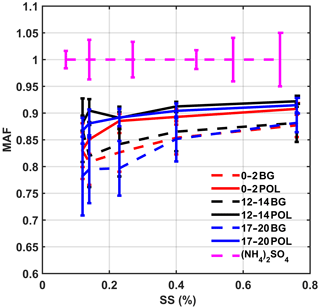Figure 5Mean maximum active fractions (MAFs) of CCN activation spectra under polluted (POL) and background (BG) conditions during the three periods of interest (00:00–02:00, 12:00–14:00, and 17:00–20:00 LT). The MAF of pure (NH4)2SO4 particles at the different SS levels (magenta line) is also plotted.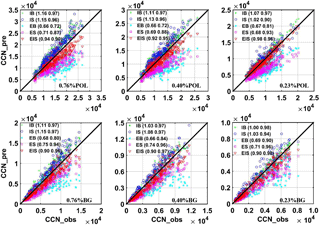Figure 6Predicted NCCN as a function of measured NCCN using the five assumptions (colored symbols – scheme associated with each symbol listed in detail at the end of this caption) at three supersaturation levels (0.23, 0.40, and 0.76 %) under polluted (POL) and background (BG) conditions. The numbers in parentheses are the slope (first number) and the correlation coefficient (second number). + INT–BK (IB) internal mixture, bulk composition;  INT–SR (IS) internal mixture, size-resolved composition; * EXT–BK (EB) external mixture, bulk composition;  EXT–SR (ES) external mixture, size-resolved composition;  EI–SR (EIS) external mixture, sulfate, nitrate, SOA, and aged BC internally mixed, and POA and fresh BC externally mixed, size-resolved composition.

### 4.2.4 CCN closure study and the sensitivity of predicted NCCN to assumed aerosol mixing state and chemical composition

Figure 6 shows the comparisons between predicted and measured NCCN at different SS levels under background and polluted conditions. The ratios of predicted-to-measured NCCN (RCCN_p∕m) ranged from 0.66 to 1.16, suggesting significant influences of the different assumptions on CCN prediction. The EI–SR assumption scheme predicts NCCN very well, with RCCN_p∕m of 0.90–0.98 (corresponding to a slight underestimation of 2–10 %). For the EI–SR scheme, hydrophobic POA and a portion of the BC are assumed to be externally mixed while the other species (sulfate, nitrate, SOA, and aged BC) are assumed to be internal mixtures. The assumption is physically sound, and the result implies that the EI–SR represents the actual mixing state and compositions of the particles well. The slight underestimation may be due to an overestimation of fresh BC caused by the method (see Sect. 2) used to retrieve it. Also, the slightly larger underestimation of NCCN for the BG case in the EI–SR scheme shown in Fig. 6 may suggest that aerosols during clean periods are mostly aged and internally mixed.

The INT–SR and INT–BK schemes which assume that aerosol is internally mixed also predict NCCN reasonably well at lower SS. The prediction is better on background days, reflecting the more homogenous aerosol composition in clean conditions. With increasing SS this overestimation became more pronounced, which is likely due to limitations of the AMS measurements. The AMS distributions show that the mass concentration was most impacted by particles with diameters near  100–400 nm. Because particles in that size range tended to be more hygroscopic than those with diameters < 100 nm, this leads to an overestimation of κ (underestimation of the critical diameter) and a resulting overestimation of NCCN at high SS. With decreasing SS the critical diameter increased and the deviation using the INT–BK and INT–SR schemes decreased. Detailed explanations about this effect have been given by Wang et al. (2010) and Zhang et al. (2017). Overall, the INT–BK and INT–SR schemes achieve CCN closure within, what is deemed here, an acceptable overprediction of 0–16 %. The EXT–BK and EXT–SR schemes underestimated NCCN, with RCCN_p∕m of 0.66 – 0.75.

Overall, the internal-mixing schemes achieve much better closure than those assuming external mixtures. Our results suggest that freshly emitted particles in urban Beijing may experience a quick conversion and mixing with pre-existing secondary particles, e.g., converting from externally mixed to internally mixed (or from hydrophobic to hydrophilic, along with a decrease in the volume of POA and BC) as previously reported (Riemer et al., 2004; Aggarwal and Kawamura, 2009; Jimenez et al., 2009; Wu et al., 2016; Peng et al., 2016). In summary, under background conditions, the INT–BK scheme achieved the best CCN closure, implying that the INT–BK assumption is likely sufficient to predict CCN in clean continental regions. However, in polluted regions, the EI–SR and INT–SR schemes may achieve better closure.

As mentioned in Sect. 2, because the SP2 measures BC core diameter and not the diameter of the BC-containing particle, the method would overestimate the BC mass concentration of smaller particles but underestimate that of larger particles. This effect adds uncertainty to the CCN prediction when using the EXT–SR scheme and is evaluated here (Fig. 7). For the evaluation, we predict NCCN with the retrieved fresh BC size distribution only in the EXT–SR scheme, which represents an upper limit of the overestimation of the fresh BC size distribution due to the SP2 measurement. Therefore, the result represents the largest underestimation of NCCN caused by the BC-containing particle effect. Our result shows that the underestimation of NCCN is reduced from 28 to 25 % by changing the total BC size distribution to that of just the fresh BC. That means that the overestimation of fresh BC due to the BC-containing particle effect in the SP2 measurements would lead to a maximum underestimation of 3 % of NCCN. The minimal uncertainty contributed by uncertainty in the BC size distribution could be explained by the small fractional contribution of BC to the total particle concentration. In conclusion, such an effect is quite small or negligible compared to the overall large underestimation of NCCN with the EXT–SR assumption.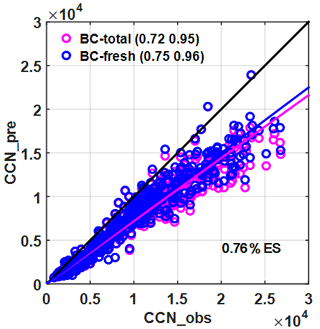Figure 7Predicted NCCN as a function of measured NCCN using the EXT–SR (ES) assumption (colored symbols) at S= 0.76 %. The pink and blue circles denote the results predicted using total and fresh BC size distributions, respectively. The numbers in parentheses are the slope (first number) and the correlation coefficient (second number).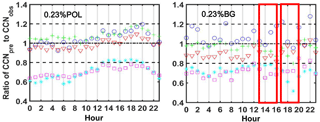Figure 8Diurnal variations in the ratio of predicted-to-measured NCCN at a supersaturation level of 0.23 % under background (BG) and polluted (POL) conditions. The following symbols are used to define the schemes displayed: + INT–BK internal mixture, bulk composition; INT–SR internal mixture, size-resolved composition; * EXT–BK external mixture, bulk composition;  EXT–SR external mixture, size-resolved composition;  EI–SR external mixture, sulfate, nitrate, SOA, and aged BC internally mixed, and POA and fresh BC externally mixed, size-resolved composition.

## 4.3 Performance of the five schemes at different times of the day

To investigate the performance of the five schemes at different times of the day, the diurnal variations in the RCCN_p∕m (SS = 0.23 %) derived by the schemes are shown in Fig. 8. In general, the INT–BK, INT–SR, and EI–SR schemes can predict NCCN well during all periods of the day under polluted or background conditions. RCCN_p∕m values are within the acceptable ±20 % uncertainty range (Wang et al., 2010; Zhang et al., 2017). Compared with other periods, the predicted NCCN during the morning and evening rush hour periods showed the most sensitivity to the different assumption schemes, especially on clean days (Fig. 8b). For example, the RCCN_p∕m derived using the INT–SR schemes reaches values up to > 1.2, and the RCCN_p∕m obtained using the EXT–BK scheme decreased to a minimum value of  0.5. The INT–SR, INT–BK, and EI–SR assumptions overestimate NCCN for the evening rush hour period by up to  20 %. This may be because most freshly emitted POA and BC particles during evening traffic hours are hydrophobic and do not contribute to the NCCN. In addition, for EI–SR assumption, a portion of BC is assumed aged and internally mixed with sulfate, nitrate, and SOA, which may reduce the actual fraction of fresh BC during rush hour period and thereby lead to an overestimation of NCCN.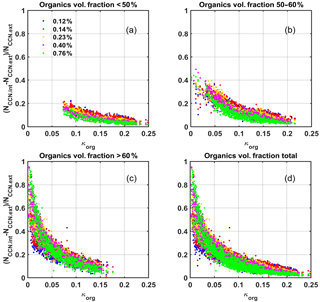Figure 9Relative deviations between NCCN predicted under the assumptions of internal (INT–BK) and external (EXT–BK) mixtures [(NCCN INT–BK NCCN EXT–BK) (NCCN EXT–BK)−1] as a function of κorg with organic volume fractions of < 50 (a), 50–60 (b), > 60 % (c) using all observed data points (d). The different colors represent different supersaturation levels (see inset in (a).

Use of the EXT–BK or EXT–SR assumption for the polluted case resulted in a predicted NCCN that was underestimated by  30–40 % at night (00:00–06:00 LT). Expectedly, the prediction using the two schemes improved during the daytime and evening rush hours, e.g., the RCCN_p∕m changed from about 0.6 to 0.8. This is likely associated with heavy urban traffic emissions/residential cooking sources during the daytime that lead to more externally mixed particles under polluted conditions; while, at night, the particles are less influenced by those local primary sources (Zhao et al., 2017). Wang et al. (2017) showed that the probability density function of κ during rush hour has a bimodal distribution and a hydrophobic mode from locally emitted particles. This also leads to reasonably accurate estimates of NCCN during nighttime with larger error during the daytime when using the internal mixing assumptions (INT–BK, INT–SR, and EI–SR) for polluted cases (Fig. 8).

## 4.4 Impact of mixing state and organic volume fraction on predicted NCCN and their variation with aerosol aging

To further examine the sensitivity of predicted NCCN to the particle mixing state and organic volume fraction with the aging of organic particles, the relative deviation between NCCN predicted assuming internal and external mixtures as a function of κorg was calculated, with the results shown in Fig. 9. The schemes that assume internal and external mixtures use bulk composition of organics, sulfate, and nitrate, which simplifies the analysis and interpretation of the results. For the data collected throughout the field campaign, the organic volume fraction is categorized as < 50, 50–60, and > 60 %. The deviation between the concentrations predicted assuming internal and external mixtures is calculated as [(NCCN INT–BK NCCN EXT–BK) (NCCN EXT–BK)−1]. The result shows that the relative deviation increased as the organic volume fraction increased. For organic volume fractions less than 50 % the maximum difference can only reach up to 20 % (SS = 0.76 %). This is consistent with previous studies that reported differences less than 20 % when xorg< 30 % (Sotiropoulou et al., 2006; Wang et al., 2010). The maximum deviation approaches 100 % for xorg > 60 % at SS = 0.76 %. Overall, the deviation is largest when the organics are less or non-hygroscopic, i.e., when κorg< 0.05. The deviation decreased rapidly as κorg increased to 0.05 in all cases. For κorg of 0.1 the differences were less than 20 %, even with high organic fractions. Moreover, differences were 10 % or less for κorg of 0.15, suggesting that the mixing state plays a minor role when κorg exceeds 0.1.

5 Conclusions

In this study, we have investigated the importance of aerosol chemical composition and mixing state on CCN activity based on measurements made during a field campaign carried out in Beijing in the winter of 2016. The NCCN was predicted by applying κ-Köhler theory and using five schemes that assume different mixing state and chemical composition combinations.

We show that there is a significant impact of the different assumptions on CCN prediction, with RCCN_p∕m ranging from 0.66 to 1.16. The best estimates of NCCN under both background and polluted conditions were obtained when using the EI–SR scheme, with a resulting RCCN_p∕m of 0.90 – 0.98. Under background conditions, the INT–BK scheme also provided reasonable estimates, with RCCN_p∕m ranging from 1.00 to 1.16. This suggests that the INT–BK assumption is likely sufficient to predict CCN in clean continental regions. On polluted days, the EI–SR and INT–SR schemes are believed to achieve better closure than the INT–BK scheme due to the heterogeneity in particle composition across different sizes. The improved closure obtained using the EI–SR and INT–SR assumptions highlights the importance of knowing the size-resolved chemical composition for CCN prediction in polluted regions. The EXT–SR and EXT–BK schemes markedly underestimate NCCN on both polluted and clean days, with an RCCN_p∕m of 0.66 – 0.75. The diurnal variations in RCCN_p∕m show that the predicted NCCN during the evening rush hour period is most sensitive to the mixing state assumptions. The RCCN_p∕m ranged from  0.5 to  1.2, reflecting the impact from evening traffic and cooking sources (both with large amounts of hydrophobic POA). We also find however, that the particle mixing state plays a minor role when κorg exceeds 0.1, even with a high organic fraction.

Data availability

The data in the study are available from the authors upon request (fang.zhang@bnu.edu.cn).

Supplement

Competing interests

The authors declare that they have no conflict of interest.

Special issue statement

Acknowledgements

This work was funded by the NSFC research project (41675141 and 91544217), the National Basic Research Program of China (2017YFC1501702), the Fundamental Research Funds for the Central Universities, and the Natural Science Foundation (NSF) (AGS1534670). We thank all participants of the field campaign for their tireless work and cooperation.

Edited by: Renyi Zhang
Reviewed by: three anonymous referees

References

Aggarwal, S. G. and Kawamura, K.: Carbonaceous and inorganic composition in long-range transported aerosols over northern Japan: Implication for aging of water-soluble organic fraction, Atmos. Environ., 43, 2532–2540, https://doi.org/10.1016/j.atmosenv.2009.02.032, 2009.

Aiken, A. C., Salcedo, D., Cubison, M. J., Huffman, J. A., DeCarlo, P. F., Ulbrich, I. M., Docherty, K. S., Sueper, D., Kimmel, J. R., Worsnop, D. R., Trimborn, A., Northway, M., Stone, E. A., Schauer, J. J., Volkamer, R. M., Fortner, E., de Foy, B., Wang, J., Laskin, A., Shutthanandan, V., Zheng, J., Zhang, R., Gaffney, J., Marley, N. A., Paredes-Miranda, G., Arnott, W. P., Molina, L. T., Sosa, G., and Jimenez, J. L.: Mexico City aerosol analysis during MILAGRO using high resolution aerosol mass spectrometry at the urban supersite (T0) – Part 1: Fine particle composition and organic source apportionment, Atmos. Chem. Phys., 9, 6633–6653, https://doi.org/10.5194/acp-9-6633-2009, 2009.

Albrecht, B. A.: Aerosols, cloud microphysics, and fractional cloudiness, Science, 245, 1227–1230, 1989.

Andreae, M. O. and Rosenfeld, D.: Aerosol–cloud–precipitation interactions. Part 1. The nature and sources of cloud-active aerosols, Earth-Sci. Rev., 89, 13–41, https://doi.org/10.1016/j.earscirev.2008.03.001, 2008.

Bhattu, D. and Tripathi, S. N.: CCN closure study: Effects of aerosol chemical composition and mixing state, J. Geophys. Res.-Atmos., 120, 766–783, https://doi.org/10.1002/2014jd021978, 2015.

Broekhuizen, K., Chang, R. Y. W., Leaitch, W. R., Li, S. M., and Abbatt, J. P. D.: Closure between measured and modeled cloud condensation nuclei (CCN) using size-resolved aerosol compositions in downtown Toronto, Atmos. Chem. Phys., 6, 2513–2524, https://doi.org/10.5194/acp-6-2513-2006, 2006.

Chang, R. Y. W., Liu, P. S. K., Leaitch, W. R., and Abbatt, J. P. D.: Comparison between measured and predicted CCN concentrations at Egbert, Ontario: Focus on the organic aerosol fraction at a semirural site, Atmos. Environ., 41, 8172–8182, 2007.

Charlson, R. J., Schwartz, S. E., Hales, J. M., Cess, R. D., Coakley Jr., J. A., Hansen, J. E., and Hofmann, D. J.: Climate forcing by anthropogenic aerosols, Science, 255, 423–430, 1992.

Che, H. C., Zhang, X. Y., Wang, Y. Q., Zhang, L., Shen, X. J., Zhang, Y. M., Ma, Q. L., Sun, J. Y., Zhang, Y. W., and Wang, T. T.: Characterization and parameterization of aerosol cloud condensation nuclei activation under different pollution conditions, Sci. Rep., 6, 24497, https://doi.org/10.1038/srep24497, 2016.

Cheng, Y. F., Su, H., Rose, D., Gunthe, S. S., Berghof, M., Wehner, B., Achtert, P., Nowak, A., Takegawa, N., Kondo, Y., Shiraiwa, M., Gong, Y. G., Shao, M., Hu, M., Zhu, T., Zhang, Y. H., Carmichael, G. R., Wiedensohler, A., Andreae, M. O., and Pöschl, U.: Size-resolved measurement of the mixing state of soot in the megacity Beijing, China: diurnal cycle, aging and parameterization, Atmos. Chem. Phys., 12, 4477–4491, https://doi.org/10.5194/acp-12-4477-2012, 2012.

Cross, E. S., Onasch, T. B., Canagaratna, M., Jayne, J. T., Kimmel, J., Yu, X. Y., Alexander, M. L., Worsnop, D. R., and Davidovits, P.: Single particle characterization using a light scattering module coupled to a time of flight aerosol mass spectrometer, Atmos. Chem. Phys., 9, 7769–7793, https://doi.org/10.5194/acp-9-7769-2009, 2009.

DeCarlo, P. F., Kimmel, J. R., Trimborn, A., Northway, M. J., Jayne, J. T., Aiken, A. C., Gonin, M., Fuhrer, K., Horvath, T., Docherty, K., Worsnop, D. R., and Jimenez, J. L.: Field-deployable, high-resolution, time-of-flight aerosol mass spectrometer, Anal. Chem., 78, 8281–8289, 2006.

Deng, Z. Z., Zhao, C. S., Ma, N., Ran, L., Zhou, G. Q., Lu, D. R., and Zhou, X. J.: An examination of parameterizations for the CCN number concentration based on in situ measurements of aerosol activation properties in the North China Plain, Atmos. Chem. Phys., 13, 6227–6237, https://doi.org/10.5194/acp-13-6227-2013, 2013.

Dusek, U., Frank, G. P., Hildebrandt, L., Curtius, J., Schneider, J., Walter, S., Chand, D., Drewnick, F., Hings, S., Jung, D., Borrmann, S., and Andreae, M. O.: Size matters more than chemistry for cloud nucleating ability of aerosol particles, Science, 312, 1375–1378, 2006.

Dzepina, K., Volkamer, R. M., Madronich, S., Tulet, P., Ulbrich, I. M., Zhang, Q., Cappa, C. D., Ziemann, P. J., and Jimenez, J. L.: Evaluation of recently proposed secondary organic aerosol models for a case study in Mexico City, Atmos. Chem. Phys., 9, 5681–5709, https://doi.org/10.5194/acp-9-5681-2009, 2009.

Ervens, B., Cubison, M., Andrews, E., Feingold, G., Ogren, J. A., Jimenez, J. L., DeCarlo, P., and Nenes, A.: Prediction of cloud condensation nucleus number concentration using measurements of aerosol size distributions and composition and light scattering enhancement due to humidity, J. Geophys. Res.-Atmos., 112, D10S32, https://doi.org/10.1029/2006JD007426, 2007.

Gunthe, S. S., King, S. M., Rose, D., Chen, Q., Roldin, P., Farmer, D. K., Jimenez, J. L., Artaxo, P., Andreae, M. O., Martin, S. T., and Pöschl, U.: Cloud condensation nuclei in pristine tropical rainforest air of Amazonia: size resolved measurements and modeling of atmospheric aerosol composition and CCN activity, Atmos. Chem. Phys., 9, 7551–7575, https://doi.org/10.5194/acp-9-7551-2009, 2009.

Gunthe, S. S., Rose, D., Su, H., Garland, R. M., Achtert, P., Nowak, A., Wiedensohler, A., Kuwata, M., Takegawa, N., Kondo, Y., Hu, M., Shao, M., Zhu, T., Andreae, M. O., and Pöschl, U.: Cloud condensation nuclei (CCN) from fresh and aged air pollution in the megacity region of Beijing, Atmos. Chem. Phys., 11, 11023–11039, https://doi.org/10.5194/acp-11-11023-2011, 2011.

Guo, S., Hu, M., Zamora, M. L., Peng, J., Shang, D., Zheng, J., Du, Z., Wu, Z., Shao, M., Zeng, L., Molina, M. J., and Zhang, R.: Elucidating severe urban haze formation in China, P. Natl. Acad. Sci. USA, 111, 17373–17378, https://doi.org/10.1073/pnas.1419604111, 2014.

Gysel, M., Crosier, J., Topping, D. O., Whitehead, J. D., Bower, K. N., Cubison, M. J., Williams, P. I., Flynn, M. J., McFiggans, G. B., and Coe, H.: Closure study between chemical composition and hygroscopic growth of aerosol particles during TORCH2, Atmos. Chem. Phys., 7, 6131–6144, https://doi.org/10.5194/acp-7-6131-2007, 2007.

Jimenez, J. L., Canagaratna, M. R., Donahue, N. M., Prevot, A. S. H., Zhang, Q., Kroll, J. H., DeCarlo, P. F., Allan, J. D., Coe, H., Ng, N. L., Aiken, A. C., Docherty, K. S., Ulbrich, I. M., Grieshop, A. P., Robinson, A. L., Duplissy, J., Smith, J. D., Wilson, K. R., Lanz, V. A., Hueglin, C., Sun, Y. L., Tian, J., Laaksonen, A., Raatikainen, T., Rautiainen, J., Vaattovaara, P., Ehn, M., Kulmala, M., Tomlinson, J. M., Collins, D. R., Cubison, M. J., Dunlea, E. J., Huffman, J. A., Onasch, T. B., Alfarra, M. R., Williams, P. I., Bower, K ., Kondo, Y., Schneider, J., Drewnick, F., Borrmann, S., Weimer, S., Demerjian, K., Salcedo, D., Cottrell, L., Griffin, R., Takami, A., Miyoshi, T., Hatakeyama, S., Shimono, A., Sun, J. Y., Zhang, Y. M., Dzepina, K., Kimmel, J. R., Sueper, D., Jayne, J. T., Herndon, S. C., Trimborn, A. M., Williams, L. R., Wood, E. C., Middlebrook, A. M., Kolb, C. E., Baltensperger, U., and Worsnop, D. R.: Evolution of organic aerosols in the atmosphere, Science, 326, 1525–1529, 2009.

Kawana, K., Nakayama, T., and Mochida, M.: Hygroscopicity and CCN activity of atmospheric aerosol particles and their relation to organics: Characteristics of urban aerosols in Nagoya, Japan, J. Geophys. Res.-Atmos., 121, 4100–4121, https://doi.org/10.1002/2015jd023213, 2016.

Lance, S., Medina, J., Smith, J., and Nenes, A.: Mapping the operation of the DMT continuous flow CCN counter, Aerosol Sci. Tech., 40, 242–254, 2006.

Li, Y., Zhang, F., Li, Z., Sun, L., Wang, Z., Li, P., Sun, Y., Ren, J., Wang, Y., Cribb, M., and Yuan, C.: Influences of aerosol physiochemical properties and new particle formation on CCN activity from observation at a suburban site of China, Atmos. Res., 188, 80–89, https://doi.org/10.1016/j.atmosres.2017.01.009, 2017.

Li, Z., Niu, F., Fan, J., Liu, Y., Rosenfeld, D., and Ding, Y.: The long-term impacts of aerosols on the vertical development of clouds and precipitation, Nat. Geosci., 4, 888–894, https://doi.org/10.1038/NGEO1313, 2011.

Liu, H. J., Zhao, C. S., Nekat, B., Ma, N., Wiedensohler, A., van Pinxteren, D., Spindler, G., Müller, K., and Herrmann, H.: Aerosol hygroscopicity derived from size-segregated chemical composition and its parameterization in the North China Plain, Atmos. Chem. Phys., 14, 2525–2539, https://doi.org/10.5194/acp-14-2525-2014, 2014.

Ma, Y., Brooks, S. D., Vidaurre, G., Khalizov, A. F., Wang, L., and Zhang, R.: Rapid modification of cloud-nucleating ability of aerosols by biogenic emissions, Geophys. Res. Lett., 40, 6293–6297, 2013.

McFiggans, G., Artaxo, P., Baltensperger, U., Coe, H., Facchini, M. C., Feingold, G., Fuzzi, S., Gysel, M., Laaksonen, A., Lohmann, U., Mentel, T. F., Murphy, D. M., O'Dowd, C. D., Snider, J. R., and Weingartner, E.: The effect of physical and chemical aerosol properties on warm cloud droplet activation, Atmos. Chem. Phys., 6, 2593–2649, https://doi.org/10.5194/acp-6-2593-2006, 2006.

Medina, J., Nenes, A., Sotiropoulou, R. E. P., Cottrell, L. D., Ziemba, L. D., Beckman, P. J., and Griffin, R. J.: Cloud condensation nuclei closure during the International Consortium for Atmospheric Research on Transport and Transformation 2004 campaign: Effects of size resolved composition, J. Geophys. Res.-Atmos., 112, D10S31, https://doi.org/10.1029/2006JD007588, 2007.

Mei, F., Setyan, A., Zhang, Q., and Wang, J.: CCN activity of organic aerosols observed downwind of urban emissions during CARES, Atmos. Chem. Phys., 13, 12155–12169, https://doi.org/10.5194/acp-13-12155-2013, 2013a.

Mei, F., Hayes, P. L., Ortega, A. M., Taylor, J. W., Allan, J. D., Gilman, J. B., Kuster, W. C., de Gouw, J. A., Jimenez, J. L., and Wang, J.: Droplet activation properties of organic aerosols observed at an urban site during CalNex-LA, J. Geophys. Res., 118, 2903–2917, https://doi.org/10.1002/jgrd.50285, 2013b.

Meng, J. W., Yeung, M. C., Li, Y. J., Lee, B. Y. L., and Chan, C. K.: Size-resolved cloud condensation nuclei (CCN) activity and closure analysis at the HKUST Supersite in Hong Kong, Atmos. Chem. Phys., 14, 10267–10282, https://doi.org/10.5194/acp-14-10267-2014, 2014.

Moore, R. H., Nenes, A., and Medina, J.: Scanning mobility CCN analysis – A method for fast measurements of size-resolved CCN distributions and activation kinetics, Aerosol Sci. Tech., 44, 861–871, https://doi.org/10.1080/02786826.2010.498715, 2010.

Moore, R. H., Cerully, K., Bahreini, R., Brock, C. A., Middlebrook, A. M., and Nenes, A.: Hygroscopicity and composition of California CCN during summer 2010, J. Geophys. Res.-Atmos., 117, D00V12, https://doi.org/10.1029/2011JD017352, 2012.

Paatero, P. and Tapper, U.: Positive matrix factorization: A non-negative factormodel with optimal utilization of error estimates of data values, Environmetrics, 5, 111–126, 1994.

Peng, J. F., Hu, M., Guo, S., Du, Z. F., Zheng, J., Shang, D. J., Zamora, M. L., Zeng, L. M., Shao, M., Wu, Y. S., Zheng, J., Wang, Y., Glen, C. R., Collins, D. R., Molina, M. J., and Zhang, R. Y.: Markedly enhanced absorption and direct radiative forcing of black carbon under polluted urban environments, P. Natl. Acad. Sci. USA, 113, 4266, https://doi.org/10.1073/pnas.1602310113, 2016.

Petters, M. D. and Kreidenweis, S. M.: A single parameter representation of hygroscopic growth and cloud condensation nucleus activity, Atmos. Chem. Phys., 7, 1961–1971, https://doi.org/10.5194/acp-7-1961-2007, 2007.

Riemer, N., Vogel, H., and Vogel, B.: Soot aging time scales in polluted regions during day and night, Atmos. Chem. Phys., 4, 1885–1893, https://doi.org/10.5194/acp-4-1885-2004, 2004.

Rose, D., Gunthe, S. S., Mikhailov, E., Frank, G. P., Dusek, U., Andreae, M. O., and Pöschl, U.: Calibration and measurement uncertainties of a continuous-flow cloud condensation nuclei counter (DMT-CCNC): CCN activation of ammonium sulfate and sodium chloride aerosol particles in theory and experiment, Atmos. Chem. Phys., 8, 1153–1179, https://doi.org/10.5194/acp-8-1153-2008, 2008.

Rose, D., Nowak, A., Achtert, P., Wiedensohler, A., Hu, M., Shao, M., Zhang, Y., Andreae, M. O., and Pöschl, U.: Cloud condensation nuclei in polluted air and biomass burning smoke near the mega-city Guangzhou, China. Part 1: Size-resolved measurements and implications for the modeling of aerosol particle hygroscopicity and CCN activity, Atmos. Chem. Phys., 10, 3365–3383, https://doi.org/10.5194/acp-10-3365-2010, 2010.

Rosenfeld, D., Lohmann, U., Raga, G. B., O'Dowd, C. D., Kulmala, M., Fuzzi, S., Reissell, A., and Andreae, M. O.: Flood or drought: How do aerosols affect precipitation?, Science, 321, 1309–1313, https://doi.org/10.1126/science.1160606, 2008.

Sotiropoulou, R.-E. P., Medina, J., and Nenes, A.: CCN predictions: Is theory sufficient for assessments of the indirect effect?, Geophys. Res. Lett., 33, L05816, https://doi.org/10.1029/2005gl025148, 2006.

Stokes, R. H. and Robinson, R. A.: Interactions in aqueous nonelectrolyte solutions. I. Solute-solvent equilibria, J. Phys. Chem., 70, 2126–2130, 1966.

Sun, J., Zhang, Q., Canagaratna, M. R., Zhang, Y., Ng, N. L., Sun, Y., Jayne, J. T., Zhang, X., Zhang, X., and Worsnop, D. R.: Highly time- and size-resolved characterization of submicron aerosol particles in Beijing using an Aerodyne Aerosol Mass Spectrometer, Atmos. Environ., 44, 131–140, https://doi.org/10.1016/j.atmosenv.2009.03.020, 2010.

Sun, Y. L., Wang, Z. F., Fu, P. Q., Yang, T., Jiang, Q., Dong, H. B., Li, J., and Jia, J. J.: Aerosol composition, sources and processes during wintertime in Beijing, China, Atmos. Chem. Phys., 13, 4577–4592, https://doi.org/10.5194/acp-13-4577-2013, 2013.

Sun, Y. L., Wang, Z. F., Du, W., Zhang, Q., Wang, Q. Q., Fu, P. Q., Pan, X. L., Li, J., Jayne, J., and Worsnop, D. R.: Long-term real-time measurements of aerosol particle composition in Beijing, China: Seasonal variations, meteorological effects, and source analysis, Atmos. Chem. Phys., 15, 10149–10165, https://doi.org/10.5194/acp-15-10149-2015, 2015.

Sun, Y. L., Chen, C., Zhang, Y., Xu, W., Zhou, L., Cheng, X., Zheng, H., Ji, D., Li, J., Tang, X., Fu, P., and Wang, Z.: Rapid formation and evolution of an extreme haze episode in Northern China during winter 2015, Sci. Rep., 6, 27151, https://doi.org/10.1038/srep27151, 2016.

Twomey, S.: The influence of pollution on the shortwave albedo of clouds, J. Atmos. Sci., 34, 1149–1152, https://doi.org/10.1175/1520-0469(1977)034<1149:TIOPOT>2.0.CO;2, 1977.

Wang, G., Zhang, R., Gomez, M. E., Yang, L., Zamora, M. L., Hu, M., Lin, Y., Peng, J. F., Guo, S., Meng, J. J., Li, J. J., Cheng, C. L., Hu, T. F., Ren, Y. Q., Wang, Y. S., Gao, J., Cao, J. J., An, Z. S., Zhou, W. J., Li, G. H., Wang, J. Y., Tian, P. F., Marrero-Ortiz, W., Secrest, J., Du, Z. F., Zheng, J., Shang, D. J., Zeng, L. M., Shao, M., Wang, W. G., Huang, Y., Wang, Y., Zhu, Y. J., Li, Y. X., Hu, J. X., Pan, B., Cai, L., Cheng, Y. T., Ji, Y. M., Zhang, F., Rosenfeld, D., Liss, P. S., Duce, R. A., Kolb, C. E., and Molina, M. J.: Persistent sulfate formation from London Fog to Chinese haze, P. Natl. Acad. Sci USA, 113, 13630–13635, 2016.

Wang, J., Flagan, R. C., and Seinfeld, J. H.: A differential mobility analyzer (DMA) system for submicron aerosol measurements at ambient relative humidity, Aerosol Sci. Tech., 37, 46–52, 2003.

Wang, J., Cubison, M. J., Aiken, A. C., Jimenez, J. L., and Collins, D. R.: The importance of aerosol mixing state and size-resolved composition on CCN concentration and the variation of the importance with atmospheric aging of aerosols, Atmos. Chem. Phys., 10, 7267–7283, https://doi.org/10.5194/acp-10-7267-2010, 2010.

Wang, Y., Zhang, F., Li, Z., Tan, H., Xu, H., Ren, J., Zhao, J., Du, W., and Sun, Y.: Enhanced hydrophobicity and volatility of submicron aerosols under severe emission control conditions in Beijing, Atmos. Chem. Phys., 17, 5239–5251, https://doi.org/10.5194/acp-17-5239-2017, 2017.

Wiedensohler, A., Cheng, Y. F., Nowak, A., Wehner, B., Achtert, P., Berghof, M., Birmili, W., Wu, Z. J., Hu, M., Zhu, T., Takegawa, N., Kita, K., Kondo, Y., Lou, S. R., Hofzumahaus, A., Holland, F., Wahner, A., Gunthe, S. S., Rose, D., Su, H., and Pöschl, U.: Rapid aerosol particle growth and increase of cloud condensation nucleus activity by secondary aerosol formation and condensation: A case study for regional air pollution in northeastern China, J. Geophys. Res.-Atmos., 114, D00G08, https://doi.org/10.1029/2008JD010884, 2009.

Wu, Y., Wang, X., Tao, J., Huang, R., Tian, P., Cao, J., Zhang, L., Ho, K.-F., Han, Z., and Zhang, R.: Size distribution and source of black carbon aerosol in urban Beijing during winter haze episodes, Atmos. Chem. Phys., 17, 7965–7975, https://doi.org/10.5194/acp-17-7965-2017, 2017.

Wu, Z. J., Zheng, J., Shang, D. J., Du, Z. F., Wu, Y. S., Zeng, L. M., Wiedensohler, A., and Hu, M.: Particle hygroscopicity and its link to chemical composition in the urban atmosphere of Beijing, China, during summertime, Atmos. Chem. Phys., 16, 1123–1138, https://doi.org/10.5194/acp-16-1123-2016, 2016.

Yum, S. S., Hudson, J. G., Song, K. Y., and Choi, B. C.: Springtime cloud condensation nuclei concentrations on the west coast of Korea, Geophys. Res. Lett., 32, L09814, https://doi.org/10.1029/2005GL022641, 2005.

Yum, S. S., Roberts, G., Kim, J. H., Song, K. Y., and Kim, D. Y.: Submicron aerosol size distributions and cloud condensation nuclei concentrations measured at Gosan, Korea, during the Atmospheric Brown Clouds East Asian Regional Experiment 2005, J. Geophys. Res.-Atmos., 112, D22S32, https://doi.org/10.1029/2006JD008212, 2007.

Zdanovskii, B.: Novyi Metod Rascheta Rastvorimostei Elektrolitovv Mnogokomponentnykh Sistema, Zh. Fiz. Khim+, 22, 1478–1495, 1948.

Zhang, F., Li, Y., Li, Z., Sun, L., Li, R., Zhao, C., Wang, P., Sun, Y., Liu, X., Li, J., Li, P., Ren, G., and Fan, T.: Aerosol hygroscopicity and cloud condensation nuclei activity during the AC3Exp campaign: Implications for cloud condensation nuclei parameterization, Atmos. Chem. Phys., 14, 13423–13437, https://doi.org/10.5194/acp-14-13423-2014, 2014.

Zhang, F., Li, Z., Li, Y., Sun, Y., Wang, Z., Li, P., Sun, L., Wang, P., Cribb, M., Zhao, C., Fan, T., Yang, X., and Wang, Q.: Impacts of organic aerosols and its oxidation level on CCN activity from measurement at a suburban site in China, Atmos. Chem. Phys., 16, 5413–5425, https://doi.org/10.5194/acp-16-5413-2016, 2016.

Zhang, F., Wang, Y., Peng, J., Ren, J., Zhang, R., Sun, Y., Collin, D., Yang, X., and Li, Z.: Uncertainty in predicting CCN activity of aged and primary aerosols, J. Geophys. Res.-Atmos., 122, 11723–11736, https://doi.org/10.1002/2017JD027058, 2017.

Zhang, Q., Worsnop, D. R., Canagaratna, M. R., and Jimenez, J. L.: Hydrocarbon-like and oxygenated organic aerosols in Pittsburgh: insights into sources and processes of organic aerosols, Atmos. Chem. Phys., 5, 3289–3311, https://doi.org/10.5194/acp-5-3289-2005, 2005.

Zhao, J., Du, W., Zhang, Y., Wang, Q., Chen, C., Xu, W., Han, T., Wang, Y., Fu, P., Wang, Z., Li, Z., and Sun, Y.: Insights into aerosol chemistry during the 2015 China Victory Day parade: results from simultaneous measurements at ground level and 260 m in Beijing, Atmos. Chem. Phys., 17, 3215–3232, https://doi.org/10.5194/acp-17-3215-2017, 2017.

Zheng, G. J., Duan, F. K., Su, H., Ma, Y. L., Cheng, Y., Zheng, B., Zhang, Q., Huang, T., Kimoto, T., Chang, D., Pöschl, U., Cheng, Y. F., and He, K. B.: Exploring the severe winter haze in Beijing: the impact of synoptic weather, regional transport and heterogeneous reactions, Atmos. Chem. Phys., 15, 2969–2983, https://doi.org/10.5194/acp-15-2969-2015, 2015.